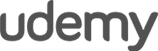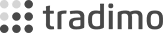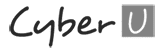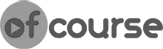Programming Market Entry Conditions – MQL4 for Complete Beginners Tutorial Part 26

Today we will continue coding the Simple System expert advisor. In this tutorial we will program the core of our Algorithmic Trading System.

We will use the OrderSend() function to send Buy and Sell orders to the market based on which way the price has moved. To check the price we will be using the Open[] array, which Metaeditor provides for us.

Source code under the video

Enrol in the full course here: https://www.forexboat.com/learn-mql4

Code for 4-digit brokers

//+------------------------------------------------------------------+
//| SimpleSystem.mq4 |
//| http://www.forexboat.com |
//+------------------------------------------------------------------+
#property version "1.00"
#property strict

extern int StartHour = 9;
extern int TakeProfit = 40;
extern int StopLoss = 40;
extern double Lots = 1;

void OnTick()
{
static bool IsFirstTick = true;
static int ticket = 0;

if(Hour() == StartHour)
{
if(IsFirstTick == true)
{
IsFirstTick = false;

if(Open < Open[StartHour])
{
ticket = OrderSend(Symbol(), OP_BUY, Lots, Ask, 10, Bid-StopLoss*Point, Bid+TakeProfit*Point, "Set by SimpleSystem");
if(ticket < 0)
{
}
}
else
{
ticket = OrderSend(Symbol(), OP_SELL, Lots, Bid, 10, Ask+StopLoss*Point, Ask-TakeProfit*Point, "Set by SimpleSystem");
if(ticket < 0)
{
}
}

}
}
else
{
IsFirstTick = true;
}

}

Code for 5-digit brokers

//+------------------------------------------------------------------+
//| SimpleSystem.mq4 |
//| http://www.forexboat.com |
//+------------------------------------------------------------------+
#property version "1.00"
#property strict

extern int StartHour = 9;
extern int TakeProfit = 40;
extern int StopLoss = 40;
extern double Lots = 1;

void OnTick()
{
static bool IsFirstTick = true;
static int ticket = 0;

if(Hour() == StartHour)
{
if(IsFirstTick == true)
{
IsFirstTick = false;

if(Open < Open[StartHour])
{
//here we are assuming that the TakeProfit and StopLoss are entered in Pips
ticket = OrderSend(Symbol(), OP_BUY, Lots, Ask, 10*10, Bid-StopLoss*Point*10, Bid+TakeProfit*Point*10, "Set by SimpleSystem");
if(ticket < 0)
{
}
}
else
{
//here we are assuming that the TakeProfit and StopLoss are entered in Pips
ticket = OrderSend(Symbol(), OP_SELL, Lots, Bid, 10*10, Ask+StopLoss*Point*10, Ask-TakeProfit*Point*10, "Set by SimpleSystem");
if(ticket < 0)
{
}
}

}
}
else
{
IsFirstTick = true;
}

}

What are you waiting for?

START LEARNING FOREX TODAY!•••••